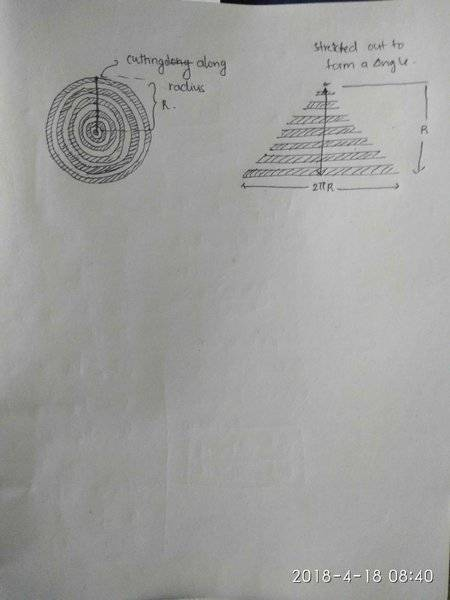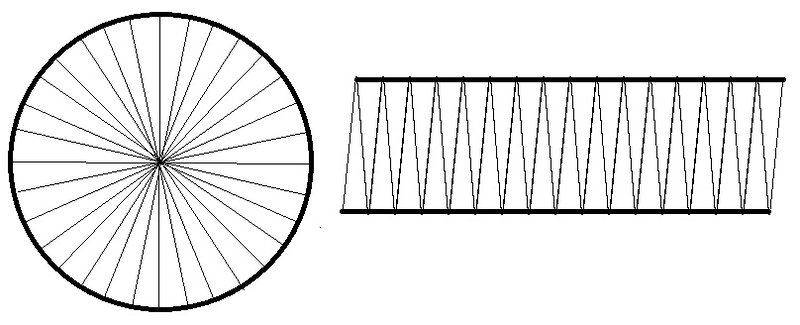# Area of a circle without calculus

• B
• Featured
π is defined by the ratio of the circumference (R) of a circle to its diameter. The area of the circle is πR². Can this be derived without calculus (or Archimedes method)?

•atyy

phyzguy
It depends what you mean by "derive" and how rigorous you want to be. If you just imagine the radius of a circle sweeping around the full circle, the outer end travels a distance of 2πr, and the center travels a distance of zero, so if you average these two you find the total area swept out is just:
$A = r * \frac{2 \pi r + 0}{2} = \pi r^2$

Of course, this is really just an intuitive application of calculus.

•Demystifier, Highwire, FallenApple and 4 others
I suppose you could make a circular field by putting a pole in the ground then dragging a string around it.

Mark44
Mentor
I suppose you could make a circular field by putting a pole in the ground then dragging a string around it.
This would determine the extent of a circle, but it wouldn't be helpful to determine its area, which is what the OP is asking about.

This would determine the extent of a circle, but it wouldn't be helpful to determine its area, which is what the OP is asking about.

We just need a steady supply of patience and 1 mm grid paper. I have a vague memory we did something like this in middle school using different shapes and 1 cm grids (counting partials as 0.5 cm2).

•256bits
Svein
Or you could use the "Monte Carlo" method - Draw two random numbers scaled to [-1, 1]. Use the first as an x-coordinate and the second as a y-coordinate. Increase a "total tries" counter. If x2+y2≤1, then increase the "inside circle" counter. Repeat...

The ratio between the "inside circle" counter and the "total tries" counter will approximate the ratio between the areas of a circle with radius 1 and a square with side 2.

•Spinnor, Biker and 256bits
All you need to do is consider there are thin circular strips inside the circle which completely fill the circle, now cut these circular rings through any one radius up to the centre and stretch them out to form a triangle.
Now,
triangle has base length =2πR
Height of the triangle =R
area (circle) = area (triangle)
= 1/2x base x height
=1/2 x 2πR x R
= πR^2

•jedishrfu and 256bits
All you need to do is consider there are thin circular strips inside the circle which completely fill the circle, now cut these circular rings through any one radius up to the centre and stretch them out to form a triangle.
Now,
triangle has base length =2πR
Height of the triangle =R
area (circle) = area (triangle)
= 1/2x base x height
=1/2 x 2πR x R
= πR^2
Construction is unclear - how do you get triangle?

Construction is unclear - how do you get triangle?
The construction is shown the document attached within this.
Hope that helps.... :)#### Attachments

•sabir and jedishrfu
It looks like a neat construction. But how you prove that the shape of the final figure is a triangle, i.e. the sides are straight lines? It looks like a geometry attempt to mimic the usual elementary calculus proof.

It looks like a neat construction. But how you prove that the shape of the final figure is a triangle, i.e. the sides are straight lines? It looks like a geometry attempt to mimic the usual elementary calculus proof.
I don't know whether it can be proved mathematically that the sides are straight lines
But, when the strips are very thin and as the radius undergoes a gradual decrease in when coming inside, so as the circumstances of inner circles also decreases the sides are going to form straight lines. My knowledge in mathematics is very primitive but, I will try to find a possible solution for this.

Mark44
Mentor
I don't know whether it can be proved mathematically that the sides are straight lines
But, when the strips are very thin and as the radius undergoes a gradual decrease in when coming inside, so as the circumstances of inner circles also decreases the sides are going to form straight lines. My knowledge in mathematics is very primitive but, I will try to find a possible solution for this.
In your first drawing in post #9, you are essentially using integration to find the area of a circle, using circular strips, or annuli.The radius of the outer circle is 1, and is centered at the origin. If we divide the circle is divided into thin concentric annuli, each of thickness ##\Delta r##, and of radius r, the area of the annulus is about ##2\pi r \cdot \Delta r##. If ##\Delta r## is reasonably "small" there's not much difference between the inner and outer radii of the annulus. In the drawing I show only one annulus, in blue.
To get an approximate value for the circle's area I can add the areas of all the annuli like so:
##A \approx \sum_{i = 1}^n 2 \pi r \Delta r##, where r is the radius to some point in the annulus, and ##\Delta r## is the thickness of the annulus, which we can take as ##\frac 1 n##.

If we make the annuli thinner (and consequently more of them), the approximation will be better. Where calculus comes in is replacing the sum above (a Riemann sum) by a definite integral: ##\int_0^1 2 \pi r dr##, which turns out, unsurprisingly, to equal ##\pi##. Unfortunately, since the OP wanted to derive the area of a circle without calculus, this solution doesn't meet that requirement.

#### Attachments

The area of a circle can be approximated without calculus but any improvement in the estimate will surely have to be made by smaller and smaller increments which eventually approach the infinitesimal. This is basically a definition of calculus.

•PeterDonis
PeterDonis
Mentor
2020 Award
the circumference (R) of a circle

If the area is ##\pi R^2##, then ##R## is the circle's radius, not its circumference.

If the area is ##\pi R^2##, then ##R## is the circle's radius, not its circumference.
My error - sorry.

π is defined by the ratio of the circumference (R) of a circle to its diameter. The area of the circle is πR². Can this be derived without calculus (or Archimedes method)?

In this source it is claimed that Gilles de Roberval, a French mathematician, was the first to accomplish to calculate the area under a sine curve without Evaluation theorem. I don't know if the same method could be applied to a circle. We might search that through the history of mathematics. The ancient Greeks could calculate it with using limits but were they the first to calculate the area of a circle?

Thanks

stevendaryl
Staff Emeritus
I have a feeling that you can't actually give a proof without calculus (or some other notion of limits), but the conclusion is made very plausible by the following construction: Divide the circle into a large number of wedges of equal size (32 are shown). Then take the wedges and rearrange them into a parallelogram. *Now, it's not actually a parallelogram, because the wedges are not exactly triangles---their short edges are slightly curved, rather than straight, but...that's why it's a plausibility argument rather than a proof). The parallelogram has a base of length ##\pi R## (since the top and bottom edges together make the full circle). The parallelogram has a height that is approximately ##r##. So the parallelogram has area ##B \cdot H = \pi R^2###### Attachments

•Demystifier, Merlin3189 and jedishrfu
Orodruin
Staff Emeritus
Homework Helper
Gold Member
I have a feeling that you can't actually give a proof without calculus (or some other notion of limits)
I agree. Essentially any "intuitive" construction by splitting the circle in smaller segments is an approximation of a Riemann sum - including of course the construction you mention later.

lavinia
Gold Member
π is defined by the ratio of the circumference (R) of a circle to its diameter. The area of the circle is πR². Can this be derived without calculus (or Archimedes method)?

Not sure what you mean by the method of Archimedes.

He did prove that the area of a circle equals the area of a right triangle whose base is the circumference and whose height is the radius. This proof does not use limits - just Euclidean geometry.

He also approximated pi which I would guess in those days was defined as half the circumference of a circle of radius one.

Last edited:
•atyy and jim mcnamara
PeterDonis
Mentor
2020 Award
He did prove that the area of a circle equals the area of a right triangle whose base is the circumference and whose height is the radius. This proof does not use limits - just Euclidean geometry.

Wikipedia has a description of Archimedes' proof here:

https://en.wikipedia.org/wiki/Area_of_a_circle#Archimedes's_proof

It appears to be a simple proof by contradiction (twice, to show that the circle's area is not greater than and not less than the triangle area).

•Demystifier
Stephen Tashi
A fundamental problem is whether we can define "area" without using concepts from calculus.

Before offering a mathematical proof about the "area" of a circle, one must have a definition of "area" that applies.

•lavinia
lavinia
Gold Member
A fundamental problem is whether we can define "area" without using concepts from calculus.

Before offering a mathematical proof about the "area" of a circle, one must have a definition of "area" that applies.

It seems like Archimedes must have realized intuitively that whatever the general definition of area may be that there must al least be inequalities. If a planar region can be covered by a figure whose area you already know then its area must be less - or if the match is exact the same. This seems to imply a limit definition.

It depends what you mean by "derive" and how rigorous you want to be. If you just imagine the radius of a circle sweeping around the full circle, the outer end travels a distance of 2πr, and the center travels a distance of zero, so if you average these two you find the total area swept out is just:
$A = r * \frac{2 \pi r + 0}{2} = \pi r^2$

Of course, this is really just an intuitive application of calculus.
I still don't see where the divided by 2 comes from. I would have thought the radius times the circumference would give the area of the circle. Clearly this isn't true, since the area of a circle is pi * r squared and it doesn't have a factor of 2 in it. Another example would be the area of a square, which is just the length of one side times the length of another side. No taking of an average involved and it makes intuitive sense.

phyzguy
With a rectangle of sides L and W, imagine taking the side of length L and 'sweeping' it across a distance W. Each end of the side moves a distance W, so it sweeps out an area of L x W. Now imagine doing the same thing with the radius of a circle, 'sweeping' it around in a 360 degree circle, with the center fixed. The center end of the radius doesn't move at all, and the outer end of the radius moves a distance 2πr. So to calculate how much area the radius sweeps out, you need to average the distance moved by the center (0) with the distance moved by the outer end (2πr), then multiply by the length of the radius (r). In calculus terms, we would write these as:$$A_{square} = \int_0^W L dx = L \times W$$
$$A_{circle} = \int_0^r 2 \pi r dr = \pi r^2$$